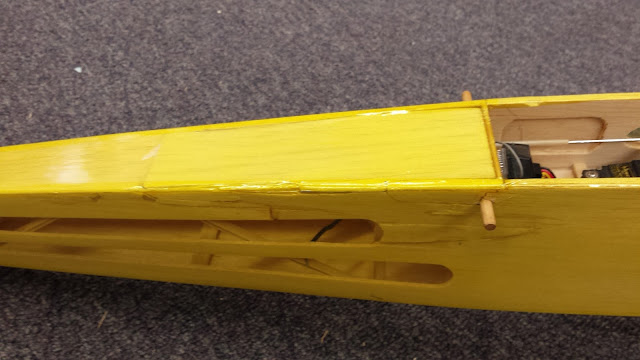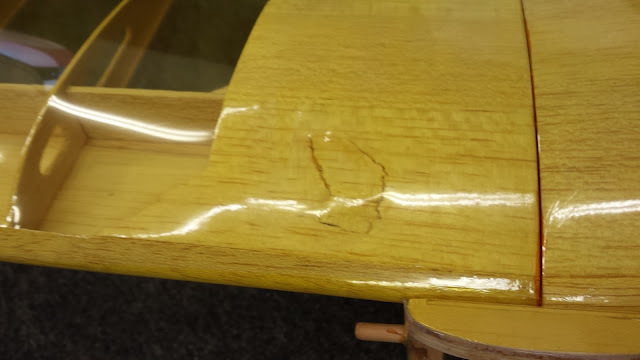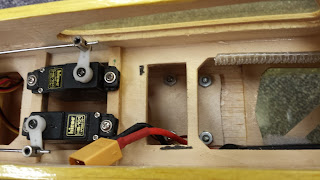## Wednesday, January 9, 2019

### Teensy Trouble

In my Ph.D. studies, I have been doing a fair amount of embedded programming. My favorite microcontroller by far has been the aptly-named Teensy. These microcontrollers have some serious hardware at a very reasonable price. They are also incredibly easy to program due to the fact that they can be programmed with arduino code. I could go on and on about why I like them, but today I found a relatively minor issue that caused me a lot of trouble.

The Teensy 3.6 has many pins capable of reading analog voltages. The two pins I decided to use for my project are pins 32 (A13) and 35 (A16). I implemented the functionality using analogRead(13)  and analogRead(16), respectively. Interestingly, A13 worked as expected, but A16 did not.  Instead, it behaved like a floating pin, and even shorting that pin to ground had no impact on the reading (this should have been a clue, actually). It wasn't just A16, either. All analog pins numbered A14 or higher were not working, while all numbered A13 or lower were working as expected.

After much frustration and much investigation, I found the answer. Since analogRead() can accept either analog pin numbers or actual pin numbers as its argument, numbers over 14 are ambiguous to the code, so it assumes the argument is the actual pin number. To avoid this, you can either use the actual pin number or use the analog pin number preceded by "A" (i.e. A14, A15, etc.). This works because those are just macros to the actual pin numbers. In my case, using analogRead(A16) solved the problem.

## Tuesday, September 27, 2016

### Predicting Control Surface Hinge Moments using Computational Fluid Dynamics

My Master's thesis has finally been published! You can view my thesis here (faster) or on The University of Alabama Electronic Theses and Dissertations database. I started this research with absolutely no CFD experience. I am quite proud of the fact that I was able to learn enough that I am now considered by many to be an expert. When I look at how far I have come in the last three years, it truly amazes me. I never thought I would learn so much.

Thanks to everyone who supported me while I worked on this research, and thanks for your continued support as I continue in my studies.

## Saturday, September 3, 2016

### Rebuilding a Three-Channel Aircraft (Dionysus)

When I was a kid, I had a 3-channel Cub-like RC airplane. While in storage, the aircraft suffered some rather severe hangar rash. The damage was the result of the unexpected compression load applied to the fuselage due to poor visibility conditions. In other words, I stepped on the plane because it was dark.

## Bringing It Back From The Dead

After I recently "retired" another aircraft when the wing incidence started varying mid-flight, I decided it was time to rebuild the old . The fuselage had been collapsed into itself, so I still had most of the broken pieces after all the years. This made reconstruction a great deal easier. Using copious amounts of wood glue and CA, and just a couple pieces of new balsa, the aircraft was ready for a new skin.

I opted to replace all of the skin because the old skin would no longer adhere to the balsa. I removed the existing skin and gave the airframe a new skin of Ultracote Lite (transparent yellow). I found that it is easy to work with, it adheres well, and it gives a very nice finish. The "Lite" version is substantially thinner than the normal stuff.The completed repair of the fuselage damage.This spot on the wing had also been damaged. The broken piece was glued back in place.

In addition to structural repairs, I decided that it was a good time to modernize the components. Gone are the 72 MHz receiver, brushed motor, gearbox, ESC, and NiMh (NiCd?) battery. I now have a Spektrum 2.4 GHz receiver, brushless motor and ESC, and a 3s LiPo battery.

I fabricated a motor mount to convert the aircraft from a brushed inrunner motor to a brushless outrunner. The entire motor mount assembly is attached to the airframe using a couple of cable ties through existing holes, so I can easily remove it if needed.  With this motor, the aircraft has much more power than it needs. The thrust-to-weight ratio is about 2, so it can climb vertically.

I also added a Mobius ActionCam to record video of my flights. I mounted the camera on the bottom of the fuselage, directly at the center of gravity. A piece of 1/8-inch birch plywood on the inside of the fuselage acts as a reinforcement plate, distributing the load to the balsa fuselage. The camera mount simply bolts onto the fuselage. You can see some footage recorded with this camera below and on my YouTube channel.You can see the reinforcement plate for the camera at the bottom of the fuselage.

The total weight of the aircraft with battery and camera installed is 1 lb 15 oz. Because I used parts that I already had lying around, the total cost of the rebuild was about \$5 plus my time. Overall, I am pleased with the quality of the rebuild.

## Test Flight Footage and Observations

Of course, the point of undertaking this repair was to get the aircraft flying again. So early one morning, I took it to the soccer field for a test flight. It turns out that 8:00 AM is a good time to fly for a number of reasons. Not only is it the most convenient time for me, but also the soccer fields are not in use, the weather is usually calm, and the lighting is perfect for filming to the west.

The aircraft itself performs very well. Since the aircraft has no ailerons, it relies on the dihedral of the wing to produce a rolling moment with rudder deflection. I mapped the rudder to the aileron channel on my transmitter. The dynamic roll response is surprisingly fast. Truth be told, I only notice the lack of ailerons during slow flight.

With the salvaged parts I had on hand, the aircraft has much more power than it needs. I estimate the thrust-to-weight ratio to be about 2, so it can climb vertically with no problem. The recorded flight lasted 10 minutes, and the 3S 2200 mAh battery was still at 11.8 V at the end of the flight.

Having successfully resurrected the aircraft, it is only appropriate to give it a suitable name. I like to name things after mythological entities, and I think Dionysus fits pretty well given the story. I just hope I don't have to change the name to Osiris down the road.

## Thursday, April 30, 2015

### Temporal and Spatial Stability Analysis of the Orr-Sommerfeld Equation

This is the second and final part of the stability analysis of the Orr-Sommerfeld Equation. In my previous post, I went through the derivation and nondimensionalization of the Orr-Sommerfeld Equation. In this part, I show how to perform the temporal and spatial stability analyses.

## Blasius Velocity Profile

A 2-D Blasius boundary layer can be expressed as
$$\bar{U}=f'\left(\eta\right)\tag{1}\label{blasius}$$
where
\begin{align}\eta=y\sqrt{\frac{U_{\infty}}{2\nu x}}\\f'''+ff''&=0\\f(0)=f'(0)&=0\\f'(\infty)&=1\end{align}
This is a nonlinear ordinary differential equation that is most easily solved using a shooting method. In a shooting method, the boundary value problem is made into an initial value problem, whereby an initial guess of one parameter is iteratively updated until the opposite boundary conditions are met. In the present case, the unknown boundary condition is $$f''(0)$$ , so it is iteratively adjusted until $$|f'(10)-1|\leq10^{-6}$$. The present case uses a fourth-order Runge-Kutta method of integration. The initial condition The transformation of the Blasius velocity profile from $$\eta$$ to $$\xi$$ is performed by determining the distance from the wall, $$\delta$$, where the stream-wise velocity is $$99.9\%$$ of the free-stream velocity.
\begin{align}\bar{U}\left(\xi\right)&=\bar{U}\left(\frac{\eta}{\delta}\right)\\\bar{U}''\left(\xi\right)&=\frac{1}{\delta^{2}}\bar{U}''\left(\frac{\eta}{\delta}\right)\end{align}\tag{2}\label{transform}
The figure below shows the Blasius velocity profile and its derivatives.

## Temporal Stability

The Orr-Sommerfeld equation can be analyzed for temporal stability by assuming that $$\bar{\alpha}$$ is real. This enables us to rearrange the non-dimensional Orr-Sommerfeld equation as follows.
$$\left(-\bar{U}\bar{\alpha}^{2}-U''+\frac{i\bar{\alpha}^{3}}{Re_{\delta}}\right)\bar{\phi}+\left(\bar{U}-\frac{2i\bar{\alpha}}{Re_{\delta}}\right)\bar{\phi}''+\left(\frac{i}{\bar{\alpha}Re_{\delta}}\right)\bar{\phi}''''=\bar{c}\left(\bar{\phi}''-\bar{\alpha}^{2}\bar{\phi}\right)\tag{3}\label{realalpha}$$
If we discretize this equation using a finite difference approximation for $$\bar{\phi}''$$ and $$\bar{\phi}''''$$, we get an equation of the form
$$A\Phi=\tilde{c}B\Phi\tag{4}\label{eigmatrixform}$$
where $$\Phi$$ is a vector containing values $$\bar{\phi}_{i}$$ at discrete locations. This is nothing more than an eigenvalue problem, where $$\tilde{c}$$ is a diagonal matrix of eigenvalues for the discrete system. The present calculations use a second order central differencing scheme to approximate the second and fourth derivative terms.
\begin{align}\bar{\phi}_{i}''&\approx\frac{\bar{\phi}_{i-1}-2\bar{\phi}_{i}+\bar{\phi}_{i+1}}{h^{2}}\\\bar{\phi}_{i}''''&\approx\frac{\bar{\phi}_{i-2}-4\bar{\phi}_{i-1}+6\bar{\phi}_{i}-4\bar{\phi}_{i+1}+\bar{\phi}_{i+2}}{h^{4}}\end{align}\tag{5}\label{eqfindiff}
At the boundaries, $$\bar{\phi}=0$$, so the first and last columns of $$A$$ and $$B$$ are removed. However, $$\bar{\phi}'=0$$ also at the boundaries. Fortunately, using a backward difference method at the boundary gives $$\bar{\phi}_{-1}\approx\bar{\phi}_{0}$$, so the $$\bar{\phi}_{i-2}$$ term can be omitted from the $$\bar{\phi}''''$$ approximation just inside the wall boundary. Similarly, the $$\bar{\phi}_{i+2}$$ term can be omitted just inside the free-stream boundary.
The finite difference approximations in Equation \ref{eqfindiff} gives a pentadiagonal $$A$$ and tridiagonal $$B$$.
\begin{align}A&=\frac{1}{h^{4}}\left[\begin{array}{ccccc}a_{11} & a_{21} & a_{3} & & 0\\a_{21} & \ddots & \ddots & \ddots\\a_{3} & \ddots & \ddots & \ddots & a_{3}\\ & \ddots & \ddots & \ddots & a_{2n}\\0 & & a_{3} & a_{2n} & a_{1n}\end{array}\right]\\B&=\frac{1}{h^{2}}\left[\begin{array}{cccc}b_{1} & b_{2} & & 0\\b_{2} & \ddots & \ddots\\ & \ddots & \ddots & b_{2}\\0 & & b_{2} & b_{1}\end{array}\right]\end{align}\tag{6}\label{fdmatrix}
where
\begin{align}a_{1i}&=h^{4}\left(-\bar{U}_{i}\bar{\alpha}^{3}-\bar{U}_{i}''\bar{\alpha}+\frac{i\bar{\alpha}}{Re_{\delta}}\right)-2h^{2}\left(\bar{U}_{i}\bar{\alpha}-\frac{2i\bar{\alpha}}{Re_{\delta}}\right)+6\left(\frac{i}{Re_{\delta}}\right)\\a_{2i}&=h^{2}\left(\bar{U}_{i}\bar{\alpha}-\frac{2i\bar{\alpha}}{Re_{\delta}}\right)-4\left(\frac{i}{Re_{\delta}}\right)\\a_{3}&=\frac{i}{Re_{\delta}}\\b_{1}&=-h^{2}\bar{\alpha}^{3}-\bar{\alpha}\\b_{2}&=\bar{\alpha}\end{align}\tag{7}\label{fdmatrixcoef}
The coefficients in $$A$$ depend on the velocity profile, which varies with the distance from the wall. However, $$B$$ is constant for a given $$\bar{\alpha}$$. In addition, $$B$$ is guaranteed to be real and symmetric, and is thus guaranteed to be invertible. We can thus left multiply Equation \ref{eigmatrixform} by $$B^{-1}$$ and rewrite the equation as follows.
$$B^{-1}A\Phi=B^{-1}\tilde{c}B\Phi\tag{8}\label{newmatform}$$
It is clear that the eigenvalues of $$B^{-1}A$$ are the eigenvalues in $$\tilde{c}$$. Matlab's eig function was used to solve for the eigenvalues for each combination of $$\bar{\alpha}$$ and $$Re$$. The following figure shows the eigenvalues of the discrete system using one value of $$\bar{\alpha}$$ and $$Re$$. Each combination of $$\bar{\alpha}$$ and $$Re$$ gives a unique set of eigenvalues similar to these.

Because this is a stability analysis, we only care about the most unstable eigenvalue. The governing equation is related to $$e^{i\alpha(x-\left(c_{r}+ic_{i}\right)t)}$$. If $$\alpha$$ is real, then any instabilities must come from an eigenvalue with a positive imaginary component. For this reason, the interesting eigenvalue is the eigenvalue with the maximum imaginary component. This analysis was done over a range of $$\bar{\alpha}$$ and $$Re$$, and the most unstable eigenvalue was stored for each combination. Matlab's contour function was then used to plot the contours shown in the following figure.

It is important to note that the contours shown here differ from those found by Wazzan in  because the Orr-Sommerfeld equation has been nondimensionalized in a different manner. The present case used the boundary layer thickness $$\delta$$, while Wazzan used displacement thickness $$\delta^{*}$$.

## Spatial Stability

The temporal stability analysis is performed assuming that $$\bar{\alpha}$$ is real. In the spatial stability analysis, we assume that $$\omega$$ is real. It is possible to derive a polynomial eigenvalue problem for the eigenvalues $$\bar{\alpha}$$, but solving the polynomial eigenvalue problem presented some challenges. Namely, at least one of the coefficient matrices was singular.

To circumvent this challenge, a mapping method has been used to give a relation between a complex $$\bar{\alpha}$$ and a complex $$\omega$$. In the present case, we hold $$\bar{\alpha}_{i}$$ at a specific value and vary $$\bar{\alpha}_{r}$$. For each $$\bar{\alpha}_{r}$$, the discrete eigenvalue problem is solved for $$\omega$$, and the most unstable eigenvalue is kept as before. By varying $$\bar{\alpha}_{r}$$ with $$\bar{\alpha}_{i}$$, a curve of $$\omega$$ values can be plotted in the complex plane, as shown in the figure above. We then determine if there is an $$\bar{\alpha}_{r}$$ that gives $$\omega_{i}=0$$ and store any locations that satisfy this condition. This process is repeated for a range of $$Re$$ for all interesting $$\bar{\alpha}_{i}$$ values. The results of this process are shown in the figure below.

## Discussion

This report is not the first on this topic, and the results do match fairly well with the references. Once the discretization was properly arranged, the eigenvalue computation was trivial using Matlab's built-in functions. Some factors added complexity to the project. The temporal stability curves are sensitive to the discretization step size and the number of $$Re$$ and $$\bar{\alpha}$$ values used. However, the spatial stability curves were far more sensitive.

It turns out that an insufficient number of $$\bar{\alpha}_{r}$$ values will give bad resolution in the complex $$\omega$$ plane, periodically overestimating the zero locations. This caused some oscillations at larger values of $$Re$$. Additionally, a large number of Reynold's Number steps were required to ensure that the each of the desired contours were visible.Compounding the issue was the fact that an extra variable effectively raised computation time by nearly an order of magnitude.

## References

1. J. D. Anderson. Fundamentals of Aerodynamics. Aeronautical and Aerospace Engineering Series. McGraw Hill, 4th edition, 2007.
2. M. J. Maghrebi. Orr Sommerfeld Solver using Mapped Finite Di fference scheme for plane wake fl ow. Journal of Aerospace Science and Technology, 2(4):55-63, December 2005
3. P. J. J. Moeleker. Linear Temporal Stability Analysis. Technical report, Delft University of Technology, 1998. Series 01: Aerodynamics 07.
4. B. S. Ng and W. H. Reid. Simple Asymptotics for the Temporal Spectrum of an Orr-Sommerfeld Problem. Applied Mathematics Letters, 13:51-55, 2000.
5. S. A. Orszag. Accurate Solution of the Orr-Sommerfeld Stability Equation. Technical report, Massachusetts Institute of Technology, May 1971.
6. T. Patel. Stability Properties of Self-propelled Wakes. Master's Thesis, University of California, San Diego, 2012.
7. A. R. Wazzan, T. T. Okamura, and A. M. O. Smith. Spatial and Temporal Stability Charts for the Falkner-Skan Boundary-layer Profiles. Technical report, Douglas Aircraft Company, 1968.
8. F. M. White. Viscous Fluid Flow. McGraw-Hill Series in Mechanical Engineering. McGraw Hill, 2nd edition, 1991.

## Matlab Codes

These are the Matlab files needed to perform this analysis. Please feel free to use them as you will, but please give me credit. A link to my blog is always appreciated.
Main Stability Analysis Code: cs_orr_sommerfeld_stability.m
Fourth Order Runge-Kutta Routine: cs_rk4.m

## Friday, April 3, 2015

### Derivation and Nondimensionalization of the Orr-Sommerfeld Equation

The Orr-Sommerfeld equation is a famous equation that can give some insight into the stability of the velocity profile of a fluid flow. This is one of two parts on the derivation and stability analysis of the Orr-Sommerfeld equation. In this post, I derive the Orr-Sommerfeld equation starting from the 2-D Navier-Stokes equations. I then show how it can be nondimensionalized. It may look like a lot of math at first glance, but it is all relatively simple.

## Derivation

The 2-D Navier-Stokes equations are given as follows:
\begin{align} \nabla\vec{V}&=0\\ \rho\frac{D\vec{V}}{Dt}&=-\nabla p+\mu\nabla^{2}\vec{V} \end{align}
Letting $$V_{x}=U+u'$$, $$V_{y}=V+v'$$, and $$p=P+p'$$ and performing a small disturbance analysis gives the small perturbation version of the Navier-Stokes Equations
\begin{align}\frac{\partial u'}{\partial x}+\frac{\partial v'}{\partial y}&=0\\ \frac{\partial u'}{\partial t}+U\frac{\partial u'}{\partial x}+V\frac{\partial u'}{\partial y}+u'\frac{\partial U}{\partial x}+v'\frac{\partial U}{\partial y}&=-\frac{1}{\rho}\frac{\partial p'}{\partial x}+\frac{\mu}{\rho}\left(\frac{\partial^{2}u'}{\partial x^{2}}+\frac{\partial^{2}u'}{\partial y^{2}}\right)\\ \frac{\partial v'}{\partial t}+U\frac{\partial v'}{\partial x}+V\frac{\partial v'}{\partial y}+u'\frac{\partial V}{\partial x}+v'\frac{\partial V}{\partial y}&=-\frac{1}{\rho}\frac{\partial p'}{\partial y}+\frac{\mu}{\rho}\left(\frac{\partial^{2}v'}{\partial x^{2}}+\frac{\partial^{2}v'}{\partial y^{2}}\right) \end{align}\tag{1}
Assuming parallel flow, where $$U\approx U(y)$$ and $$V\approx0$$, we can simplify this to the the following form of the Navier-Stokes equations.
\begin{align}\frac{\partial u'}{\partial x}+\frac{\partial v'}{\partial y}&=0\\\frac{\partial u'}{\partial t}+U\frac{\partial u'}{\partial x}+v'\frac{\partial U}{\partial y}&=-\frac{1}{\rho}\frac{\partial p'}{\partial x}+\frac{\mu}{\rho}\left(\frac{\partial^{2}u'}{\partial x^{2}}+\frac{\partial^{2}u'}{\partial y^{2}}\right)\\\frac{\partial v'}{\partial t}+U\frac{\partial v'}{\partial x}&=-\frac{1}{\rho}\frac{\partial p'}{\partial y}+\frac{\mu}{\rho}\left(\frac{\partial^{2}v'}{\partial x^{2}}+\frac{\partial^{2}v'}{\partial y^{2}}\right)\end{align}\label{simp_NS}\tag{2}
In this analysis, disturbances are assumed to be Tollmien-Schlichting waves, with the general form as follows.
\begin{align}\psi&=\phi(y)e^{i(\alpha x-\omega t)}\\u'&=\frac{\partial\psi}{\partial y}=\frac{\partial\phi}{\partial y}e^{i(\alpha x-\omega t)}\\v'&=-\frac{\partial\psi}{\partial x}=-i\alpha\phi e^{i(\alpha x-\omega t)}\end{align}\tag{3}
The temporal and spatial derivatives are then calculated as follows.
\begin{align}\frac{\partial u'}{\partial t}&=-i\omega\frac{\partial\phi}{\partial y}e^{i(\alpha x-\omega t)}\\\frac{\partial u'}{\partial x}&=i\alpha\frac{\partial\phi}{\partial y}e^{i(\alpha x-\omega t)}\\\frac{\partial^{2}u'}{\partial x^{2}}&=-\alpha^{2}\frac{\partial\phi}{\partial y}e^{i(\alpha x-\omega t)}\\\frac{\partial u'}{\partial y}&=\frac{\partial^{2}\phi}{\partial y^{2}}e^{i(\alpha x-\omega t)}\\\frac{\partial^{2}u'}{\partial y^{2}}&=\frac{\partial^{3}\phi}{\partial y^{3}}e^{i(\alpha x-\omega t)}\\\frac{\partial v'}{\partial t}&=-\alpha\omega\phi e^{i(\alpha x-\omega t)}\\\frac{\partial v'}{\partial x}&=\alpha^{2}\phi e^{i(\alpha x-\omega t)}\\\frac{\partial^{2}v'}{\partial x^{2}}&=i\alpha^{3}\phi e^{i(\alpha x-\omega t)}\\\frac{\partial v'}{\partial y}&=-i\alpha\frac{\partial\phi}{\partial y}e^{i(\alpha x-\omega t)}\\\frac{\partial^{2}v'}{\partial y^{2}}&=-i\alpha\frac{\partial^{2}\phi}{\partial y^{2}}e^{i(\alpha x-\omega t)}\end{align}\tag{4}
We can then substitute each of these derivatives into Equation $$\ref{simp_NS}$$ and we get the following relations.
\begin{align}e^{i(\alpha x-\omega t)}\left[i\alpha\frac{\partial\phi}{\partial y}-i\alpha\frac{\partial\phi}{\partial y}\right]&=0\\-\rho e^{i(\alpha x-\omega t)}\left[-i\omega\frac{\partial\phi}{\partial y}+i\alpha U\frac{\partial\phi}{\partial y}+-i\alpha\phi\frac{\partial U}{\partial y}-\frac{\mu}{\rho}\left(-\alpha^{2}\frac{\partial\phi}{\partial y}+\frac{\partial^{3}\phi}{\partial y^{3}}\right)\right]&=\frac{\partial p'}{\partial x}\\-\rho e^{i(\alpha x-\omega t)}\left[-\alpha\omega\phi+U\alpha^{2}\phi-\frac{\mu}{\rho}\left(i\alpha^{3}\phi-i\alpha\frac{\partial^{2}\phi}{\partial y^{2}}\right)\right]&=\frac{\partial p'}{\partial y}\end{align}
To eliminate the pressure fluctuation term, differentiate the x- and y-momentum equations by $$y$$ and $$x$$, respectively.
\begin{align}\frac{1}{-\rho e^{i(\alpha x-\omega t)}}\frac{\partial^{2}p'}{\partial x\partial y}&=-i\omega\frac{\partial^{2}\phi}{\partial y^{2}}+i\alpha\frac{\partial U}{\partial y}\frac{\partial\phi}{\partial y}+i\alpha U\frac{\partial^{2}\phi}{\partial y^{2}}-i\alpha\frac{\partial\phi}{\partial y}\frac{\partial U}{\partial y}\\&\quad-i\alpha\phi\frac{\partial^{2}U}{\partial y^{2}}+\frac{\mu}{\rho}\left(\alpha^{2}\frac{\partial^{2}\phi}{\partial y^{2}}-\frac{\partial^{4}\phi}{\partial y^{4}}\right)\\\frac{1}{-i\alpha\rho e^{i(\alpha x-\omega t)}}\frac{\partial^{2}p'}{\partial x\partial y}&=-\alpha\omega\phi+U\alpha^{2}\phi+\frac{\mu}{\rho}\left(-i\alpha^{3}\phi+i\alpha\frac{\partial^{2}\phi}{\partial y^{2}}\right)\end{align}\tag{5}
Equating the two momentum equations gives
$$-i\omega\frac{\partial^{2}\phi}{\partial y^{2}}+i\alpha U\frac{\partial^{2}\phi}{\partial y^{2}}-i\alpha\phi\frac{\partial^{2}U}{\partial y^{2}}+\frac{\mu}{\rho}\left(2\alpha^{2}\frac{\partial^{2}\phi}{\partial y^{2}}-\frac{\partial^{4}\phi}{\partial y^{4}}-\alpha^{4}\phi\right)+i\alpha^{2}\omega\phi-iU\alpha^{3}\phi=0$$
This simplifies to the Orr-Sommerfeld Equation.
$$\left(U-\frac{\omega}{\alpha}\right)\left(\frac{\partial^{2}\phi}{\partial y^{2}}-\alpha^{2}\phi\right)-\phi\frac{\partial^{2}U}{\partial y^{2}}+\frac{i\nu}{\alpha}\left(\frac{\partial^{4}\phi}{\partial y^{4}}-2\alpha^{2}\frac{\partial^{2}\phi}{\partial y^{2}}+\alpha^{4}\phi\right)=0\label{orrsommerfeld}\tag{6}$$

## Nondimensionalization

The Orr-Sommerfeld equation is nondimensionalized using the following nondimensional parameters,
$$\bar{U}=\frac{U}{U_{\infty}}\quad\xi=\frac{y}{\delta}\quad\bar{\phi}=\frac{\phi}{U_{\infty}\delta}\quad\bar{c}=\frac{c}{U_{\infty}}\quad\bar{\alpha}=\alpha\delta\quad Re_{\delta}=\frac{U_{\infty}\delta}{\nu}\tag{7}$$
where $$c=\frac{\omega}{\alpha}$$ and $$\delta$$ is the boundary layer thickness. Substituting these into Equation $$\ref{orrsommerfeld}$$ gives
\begin{align}0&=\left(\bar{U}U_{\infty}-\bar{c}U_{\infty}\right)\left(\frac{1}{\delta^{2}}\frac{\partial^{2}}{\partial\xi^{2}}\left(\bar{\phi}U_{\infty}\delta\right)-\left(\frac{\bar{\alpha}}{\delta}\right)^{2}\left(\bar{\phi}U_{\infty}\delta\right)\right)-\frac{\bar{\phi}U_{\infty}\delta}{\delta^{2}}\frac{\partial^{2}}{\partial\xi^{2}}\left(\bar{U}U_{\infty}\right)\\&\quad+\frac{i\nu\delta}{\bar{\alpha}}\left(\frac{1}{\delta^{4}}\frac{\partial^{4}}{\partial\xi^{4}}\left(\bar{\phi}U_{\infty}\delta\right)-\frac{2\bar{\alpha}^{2}}{\delta^{4}}\frac{\partial^{2}}{\partial\xi^{2}}\left(\bar{\phi}U_{\infty}\delta\right)+\frac{\bar{\alpha}^{4}}{\delta^{4}}\left(\bar{\phi}U_{\infty}\delta\right)\right)\end{align}\tag{8}
Applying the chain rule for each partial derivative gives
\begin{align}0&=U_{\infty}\left(\bar{U}-\bar{c}\right)\left(\frac{U_{\infty}}{\delta}\frac{\partial^{2}\bar{\phi}}{\partial\xi^{2}}-\frac{\bar{\alpha}^{2}U_{\infty}}{\delta}\bar{\phi}\right)-\frac{U_{\infty}^{2}}{\delta}\frac{\partial^{2}\bar{U}}{\partial\xi^{2}}\bar{\phi}\\&\quad+\frac{i\nu\delta}{\bar{\alpha}}\left(\frac{U_{\infty}}{\delta^{3}}\frac{\partial^{4}\bar{\phi}}{\partial\xi^{4}}-\frac{2\bar{\alpha}^{2}U_{\infty}}{\delta^{3}}\frac{\partial^{2}\bar{\phi}}{\partial\xi^{2}}+\frac{\bar{\alpha}^{4}U_{\infty}}{\delta^{3}}\bar{\phi}\right)\end{align}\tag{9}
Finally, factor out $$\frac{U_{\infty}^{2}}{\delta}$$ and substitute for $$Re_{\delta}$$ to get
$$\left(\bar{U}-\bar{c}\right)\left(\frac{\partial^{2}\bar{\phi}}{\partial\xi^{2}}-\bar{\alpha}\bar{\phi}\right)-\frac{\partial^{2}\bar{U}}{\partial\xi^{2}}\bar{\phi}+\frac{i}{\bar{\alpha}Re_{\delta}}\left(\frac{\partial^{4}\bar{\phi}}{\partial\xi^{4}}-2\bar{\alpha}^{2}\frac{\partial^{2}\bar{\phi}}{\partial\xi^{2}}+\bar{\alpha}^{4}\bar{\phi}\right)=0\tag{10}$$
For convenience, derivatives with respect to the station coordinate $$\xi$$ are hereafter denoted with prime notation. This gives the final nondimensional form of the Orr-Sommerfeld equation:
$$\left(\bar{U}-\bar{c}\right)\left(\bar{\phi}''-\bar{\alpha}\bar{\phi}\right)-\bar{U}''\bar{\phi}+\frac{i}{\bar{\alpha}Re_{\delta}}\left(\bar{\phi}''''-2\bar{\alpha}^{2}\bar{\phi}''+\bar{\alpha}^{4}\bar{\phi}\right)=0\tag{11}$$